Finally, a Math Education Tool that Supports Research-Based Learning Theory!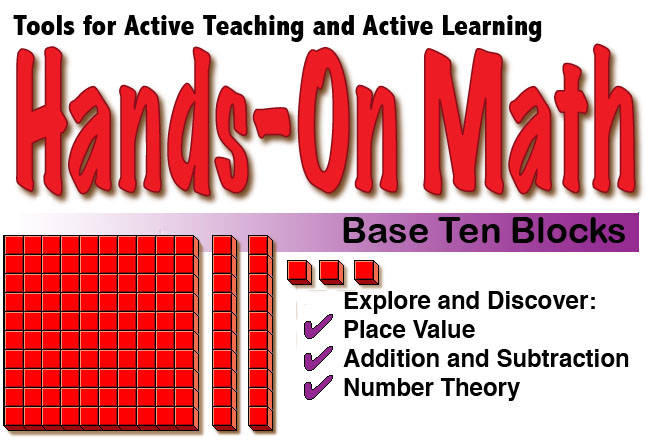Base Ten Blocks are a very useful way for student visualize images of numbers, intuitively understand place value and deepen their understanding of addition and subtraction. Use Base Ten Blocks to have students represent numbers, as well as read and write the numbers. Rules for regrouping can be difficult for children. Use Base Ten Blocks to illustrate regrouping by dragging and dropping blocks from one column to another. Hands-On Math: Base Ten Blocks simulates the use of a popular math manipulative used to teach place value and addition and subtraction with regrouping. Base Ten Blocks are one of the best ways to help students place value. Students place Base Ten Blocks on an interactive Playground to represent numbers. Blocks can be regrouped or exchanged by dragging a virtual manipulative from one column to the next. Using Hands-On Math: Base Ten Blocks students can develop the following important mathematical concepts: Place value concepts Regrouping in the ones, tens and hundreds places Basic operations: addition and subtraction Naming numbers Expanded notation Reading and writing numbersWe recommend that you open the Instructor's Guide PDF from your iPad in a new browser page and save the document in your iBooks app.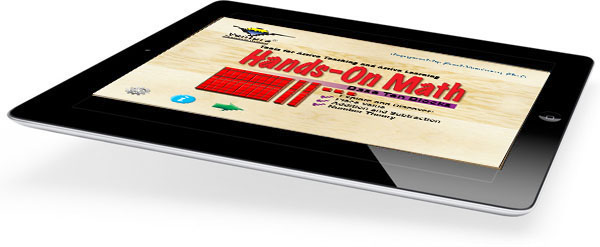Features: Open-ended Interactive Base Ten Blocks Playground. Supports Discovery and Directed Teaching Strategies . Problem Solving Activities which Can Be Solved Using Virtual Manipulatives. Base Ten Blocks Help to Build a Fundamental Math Concepts. On/Off Options and Controls for Various Features. \$2.99
 Base Ten Blocks Base Ten Blocks are used to represent numbers. Three types of blocks are used Hundreds, Tens and Ones. Possible Topics: Place Value Addition Subtraction Regrouping Decimals Naming, reading and writing numbers. Expanded Notation.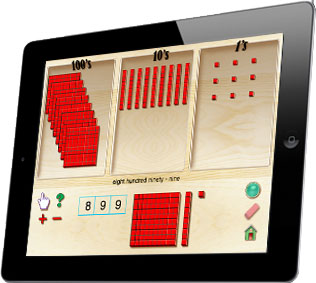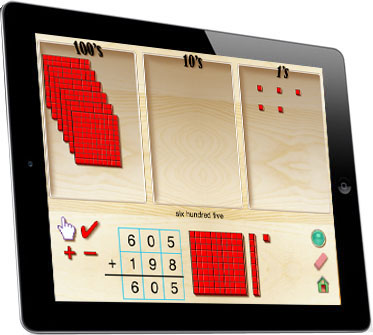Base Ten Blocks By tapping an icon a randomly generated problem appears on the screen. Using Base Ten Blocks students enter the answer to the problem. If an error occurs, specific feedback about the error is given. Explore place value. Practice addition and subtraction skills. Learn when regrouping is needed.Explore and Document Activities at a variety of skill levels are also provided. Students are encouraged to document their discoveries in a journal. Here the students are asked to record the problems and answer generated in the quiz to practice reading and writing numbers as well as other important math skills. Perfect for Small Group instruction Environmentally Friendly Learning - (no need to photocopy activity pages for each student) A Student-Centered Approach to Learning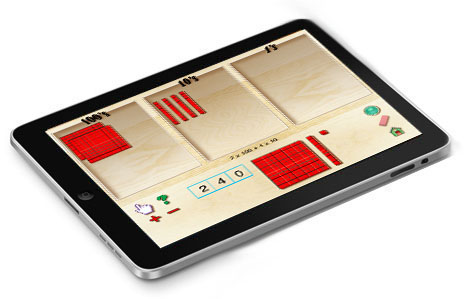Hands-On Math: Base Ten Blocks is now available at the Apple iTunes Store.